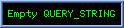Most of the multimeters do not offer resistance measurement in the milliohm range. In most meters a typically Ohm range has an accuracy of 0.1 ohm and is too coarse for measuring small resistance. Of course, some of the high-end multimeters have milliohm range and there are also dedicated micro ohmmeters for even more accurate small resistance measurements, but most of them are priced way out of the range for personal use. The key in resistance measurement is nothing more than Ohm s law. And any unknown resistance can be calculated by using either a known voltage source and measure the current or a known current source and measure the voltage drop across the resistance to be measured. Typically, small resistance in the milliohm range is measured using the 4-point Kelvin sensing method to eliminate wiring resistance of the probes and thus makes the measurement more accurate. One of the problems of measuring small resistance is that for a given current value, as the resistance to be measured goes lower, the voltage drop becomes smaller as well and in most of the cases this voltage drop becomes too small to be measured accurately. For instance the voltage generated across a 10 milli-ohm resistor from 100 mA current is only 1 mV, which is too small for most multimeters. Although the issue mentioned above can be somewhat resolved by increasing the current passed through the resistor, there is an other issue associated with this approach. Unfortunately, the heat generated from the excessive current flow affects the accuracy of the measurement. And sometimes, the target to be measured simply cannot handle current this high. To effectively measure small resistance values in the milliohm range, we need to accurately measure the current flowing through and at the same time accurately capture the minute voltage drop across the terminals. To accurately measure small voltages, one can use a programmable-gain precision instrumentation operational amplifier such as National Semiconductor s LMP8358. While you can use other op-amps with varies gains, one of the key benefits of using a programmable-gain gain precision instrumentation op-amp is that there is no need to match the gain setting resistors. LMP83582 s gain can be programmatically set to 10, 20, 50, 100, 200, 500 and 1000, which is superb for amplifing the millivolts voltage drop. An accurate constant current source is needed to generate a known current reference. We could use an additional multimeter to measure the current and thus eliminating the need for a current source, but it would be too cumbersome for regular use. For the ease of metering, it is usually a good idea to set the current source so that the current and voltage product is a power of 10. For instance, for a gain of 100, setting the current to 10 mA seems to be a good compromise between the current flow and the overall accuracy. The resultant measurement readings will be one 1mV per milliohm which is quite convenient. With this setting, you should be able to measure accurately down to 10 milliohm (10 mV reading). And by setting the gain to 1000 (10 mV/milliohm) we can measure resistance all the way down to 1 milliohm. The circuit for accurate milliohm measurement is shown below. It basically is a 10 mA precision current source followed by an zero-offset instrumentation amplifier with a gain of 100.Accurate Milliohm Measurement

by Kerry WONG (original here)